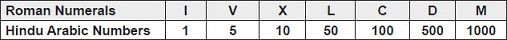# User ForumSubject :IMO    Class : Class 4

Using the given table and answer the following questions.CM = ____.

A 100 - 1000
B 1000 - 100
C 500 - 100
D 1000 - 10

It is a very tricky question

## Ans 1:

Class : Class 5
The correct answer is b

Class : Class 5
a

Class : Class 6
A

Class : Class 2
CM=1000-100

## Ans 5:

Class : Class 9
B is the correct answer because we cannot subtract 1000 from 100

Class : Class 4
b is the answer

## Ans 7:

Class : Class 6
The correct option feeded into the question is wrong. It should be C, but the correct answer in this is B!

## Ans 8:

Class : Class 9
c=100,M=1000 so cm =100-1000 (answer. c)

## Ans 9:

Class : Class 6
The correcr answer is B CM = 1000 – 100.

## Ans 10:

Class : Class 4
If there is C before M that means that we are subtracting 100 from 1000 . We write it as 1000 - 100. Answer : B

Class : Class 6
A

## Ans 12: (Master Answer)

Class : Class 1

The correcr answer is B

CM = 1000 – 100.

## Ans 13:

Class : Class 7
The answer is B because CM=C-M=C(100)-M(1000)We cannot subtract 100-1000 So the formula should be 1000-100 l Therefore, TH H T O 1 0 0 0 - 1 0 0 ----------------------- 9 0 0 So, B is the right answer.

## Ans 14:

Class : Class 3
The correct answer is option B.

Class : Class 4

## Ans 16:

Class : Class 5
The correct answer would be option C because CM = 900. C=100, M=1000. So, the smaller no. is in the beginning, it will be minus. So, 1000-100 is the correct ans.

Class : Class 5
B

## Ans 18:

Class : Class 1
bakwaas question hai

Class : Class 5
B